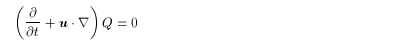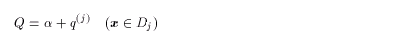2. Theory
2.1 Contour Dynamics
2.2 Equations of Cotour Dynamics
2.3 Theory of an Elliptical Vortex
2.4 Finite-Time Lyapunov Analysis on Fluid Deformation

2.1 Contour Dynamics

Motion in two-dimensional nondivergent perfect fluid can be described using Lagrangian conservation law of vorticity:(2.1)
where u( x,t ) is the velocity, and Q( x,t ) is the vertical component of the vorticity. The vorticity distribution is assumed to be(2.2)
for regions Dj ( j=1,2, ... ,J ) ( Dj1ÇDj2 = j ) on an infinite plane. Each region is called vortex patch.

In this situation,

• The vorticity in each region keeps constant in time due to Lagrangian conservation law.
• Thus time evolution of the system can be obtained by chasing the position of the contours (boundary lines) of the regions.
• Time evolution of the contour positions can be calculated from the contour positions themselves, because the velocity at an arbitrary point can be calculated by them.
• As a result, time evolution of the system is described as the contour deformation.
Such dynamics concerning time evolution of the vortex patch is called contour dynamics.